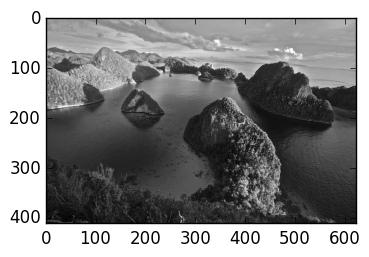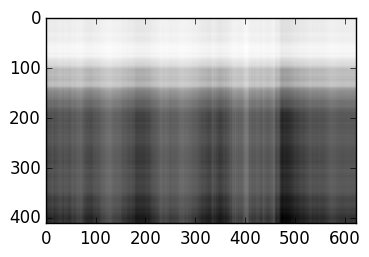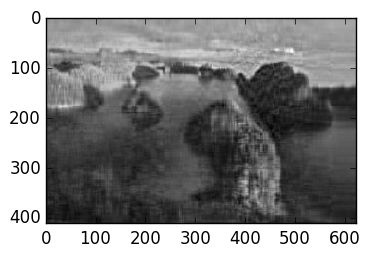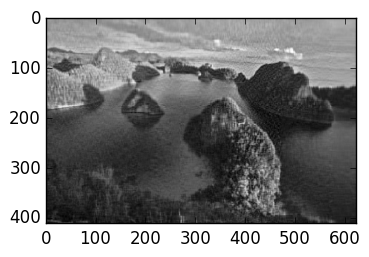# Lecture 22: The Singular Value Decomposition (SVD)¶

This lecture introduces the singular value decomposition, which we will use to compress images.

# SVD¶

The SVD is a decomposition of an $n \times m$ matrix $A$ as

$$A = U \Sigma V^\top$$

where $U \in \mathbb R^{n\times n}$ and $V \in \mathbb R^{m\times m}$ are orthogonal and $\Sigma \in \mathbb R^{n \times m}$ is diagonal, of the form ($n \geq m$)

$$\Sigma = \begin{pmatrix} \sigma_1 \cr & \sigma_2 \cr &&\ddots \cr &&& \sigma_m \cr &&& 0 \cr &&&\vdots \cr &&&0\end{pmatrix},$$

where $\sigma_1 \geq \sigma_2 \geq \cdots \geq \sigma_m \geq 0$.

The SVD can be calculated using the svd command:

In :
A=rand(5,5)
U,σ,V=svd(A)

Σ=diagm(σ)

norm(A-U*Σ*V')

Out:
2.0261379083813132e-15

U and V are indeed orthogonal:

In :
norm(U'*U-I),norm(V'*V-I)

Out:
(1.3615860578367606e-15,6.210964166796445e-16)

## Relationship with eigenvalue decomposition¶

The SVD is closely connected to the eigenvalue decomposition for symmetric matrices. The difference is that eigenvalues are signed while singular values are all positive:

In :
A=rand(5,5)
A=A+A'       #   any matrix plus its transpose is symmetric
svdvals(A),eigvals(A)  # the magnitudes are the same, but the sign and ordering are differend

Out:
([5.169732137294604,1.6312252622180625,1.0174977889866161,0.8976829470592561,0.7363935801508221],[-1.0174977889866166,-0.8976829470592556,0.7363935801508223,1.6312252622180614,5.169732137294606])

A symmetric matrix has an eigenvalue decomposition

$$A = Q \Lambda Q^\top$$

where $Q$ is orthogonal (we'll explain this in two lectures):

In :
λ,Q=eig(A)
Λ=diagm(λ)
norm(A-Q*Λ*Q')

Out:
5.706584266746393e-15

For symmetric matrices, singular values are the absolute value of eigenvalues. We can see this by constructing the SVD from an eigenvalue decomposition by permuting and taking the absolute value:

In :
P=eye(5)[:,[5,4,1,3,2]]

norm(A-Q*P*P'*abs(Λ)*P*P'*sign(Λ)*Q')

Ū=Q*P
Σ̄=P'*abs(Λ)*P
V̄=Q*sign(Λ)*P

norm(Ū*Σ̄*V̄'-A)

Out:
5.632251628873568e-15

Singular values are not (necessarily) absolute value of eigenvalues for non-symmetric matrices: in the following matrix, the eigenvalues are zero but the singular values are not:

In :
A=[0 1.0;
0  0]

svdvals(A)  # returns σ, the list of singular values

Out:
2-element Array{Float64,1}:
1.0
0.0

# Properties of the SVD:¶

1) The 2-norm of a matrix is the largest singular value:

$$\|A\|_2 = \sigma_1:$$
In :
A=rand(5,3)
U,σ,V=svd(A)
maximum(σ),norm(A)

Out:
(2.0999467726097785,2.099946772609779)

This follows since orthogonal matrices do not change 2-norms:

$$\|A\|_2 \triangleq \sup_{\|\mathbf w \| = 1} \|A \mathbf w\| = \sup_{\|\mathbf w \| = 1} \|U \Sigma V^\top \mathbf w\| = \sup_{\|\mathbf w \| = 1} \|U \Sigma V^\top \mathbf w\| = \sup_{\|\mathbf v \| = 1} \| \Sigma \| = \max_k{\sigma_k} = \sigma_1$$

2) The rank of a matrix is the number of non-zero singular values

This follows since the rank of a diagonal matrix (in this case $\Sigma$) is the number of non-zero entries, and $U$ and $V$ are of full rank.

3) The kernel of a rank-r matrix is the span of V[:,r+1:end].

This follows since:

$$A \mathbf v_k = U \Sigma V^\top V \mathbf e_k = U \Sigma \mathbf e_k = 0$$

if $k > r$.

#### The best rank-r approximation¶

Given the SVD, the best rank-r approximation is given by dropping all but the first r singular values:

$$A_r \triangleq U \Sigma_r V^\top$$

where

$$\Sigma_r \triangleq \begin{pmatrix} \sigma_1 \cr & \sigma_2 \cr &&\ddots \cr &&& \sigma_r \cr &&&& 0 \cr &&&&& \ddots \cr &&&&&& 0 \cr &&&&&&\vdots \cr &&&&&&0\end{pmatrix},$$

Theorem $A_r$ is the best 2-norm approximation of $A$ by a rank $r$ matrix, that is, for all rank-$r$ matrices $B$, we have

$$\|A - A_r\|_2 \leq \|A -B \|_2.$$

Proof We have

$$\|A - A_r\|_2 = \|U \begin{pmatrix} 0 \cr &\ddots \cr && 0 \cr &&& \sigma_{r+1} \cr &&&& \ddots \cr &&&&& \sigma_n \cr &&&&&\vdots \cr &&&&&0\end{pmatrix} = \sigma_{r+1}$$

Suppose a rank-$r$ matrix $B$ has

$$\|A-B\|_2 < \|A-A_r\|_2 = \sigma_{r+1}.$$

For all $\mathbf w \in \ker(B)$ we have

$$\|A \mathbf w\|_2 = \|(A-B) \mathbf w\|_2 \leq \|A-B\|\|\mathbf w\|_2 < \sigma_{r+1} \|\mathbf w\|_2$$

But for all $\mathbf w \in {\rm span}\, V[1:r+1]$, that is, $\mathbf w = V[1:r+1]\mathbf c$ for some $\mathbf c \in \mathbb R^{r+1}$ we have

$$\|A \mathbf w\|_2^2 = \|U \Sigma[:,1:r] \mathbf c\|_2^2 = \|\Sigma[:,1:r] \mathbf c\|_2^2 = \sum_{k=1}^{r+1} (\sigma_k c_k)^2 \geq \sigma_{r+1}^2 \|c\|^2,$$

i.e., $\|A \mathbf w\|_2^2 \geq \sigma_{r+1} \|c\|$.

The dimension of the span of $\ker(B)$ is at least $n-r$, but the dimension of ${\rm span}\, V[1:r+1]$ is at least $r+1$. Since these two spaces cannot intersect we have a contradiction, since $(n-r) + (r+1) = n+1 > n$. ∎

## Application: image compression¶

We'll see an application of this to image compression: we are going to approximate an image $A$ by its best rank-$r$ approximation $A_r$. First load up a (greyscale) image:

In :
using PyPlot

imshow(img)

R=img[:,:,1]

A=1-R  # swap white and black

imshow(A;cmap=:Greys);The following gives the best rank-1 approximation:

In :
U,σ,V=svd(A)

imshow(U[:,1]*σ*V[:,1]';cmap=:Greys);Taking more and more singular values gives higher rank approximation:

In :
U,σ,V=svd(A)

r=20
imshow(U[:,1:r]*diagm(σ[1:r])*V[:,1:r]';cmap=:Greys);In :
U,σ,V=svd(A)

r=50
imshow(U[:,1:r]*diagm(σ[1:r])*V[:,1:r]';cmap=:Greys);We can store the resulting image using just the matrices U[:,1:r]*diagm(σ[1:r]) and V[:,1:r], for a total of $rn + rm$ floating point numbers. For r=20, this is only about 20% of the storage as storing every pixel:

In :
n,m=size(A)
(r*n+r*m)/(n*m)   # We use only 20% of the data as the full matrix

Out:
0.20174507539100303